## Shapley Galaxy Set¶

version 1.0 7/3/2018 Wing-Fai Thi

### Astronomical backgroud:¶

• The distribution of galaxies in space is strongly clustered.
• These clusters form Large Scale Structure (LSS) of the Universe.
• The clustering is hierarchical, nonlinear, and anisotropic.
• Galaxies are concentrated in giant flattened, curved superclusters surrounding "voids".
• The gravitational attraction of matter in the Universe expanding from the Big Bang ~14 billion years ago creates the structure.
• The pattern is well reproced by advanced numerical simulation taking into account attractive Cold Dark Matter and repulsive Dark Energy into addition to the baryonic (normal) matter.
• Questions remain on the processes of collision and merging of rich galaxy clusters

### Dataset:¶

• CASt dataset made availalbe by Michael Drinkwater, University of Queensland
• The Shapley Supercluster or Shapley Concentration (SCl 124) is the largest concentration of galaxies in our nearby universe that forms a gravitationally interacting unit
• Redshifts (i.e. velocities in km/s with respect to us) are now measured for 4215 galaxies in the Shapley Concentration regions (Drinkwater et al. 2004).

### References:¶

• Modern Statistical Methods for Astronomy E. Feigelson & J. Babu, Cambdridge Univeristy Press
• scikit-learn user guide, see the section "Comparing different clustering algorithms on toy datasets"
• Statistics, Data Mining, and Maching Learning in astronomy Ivezic et al. Princeton Series in Modern Observational Astronomy

### Aim of the notebooks:¶

The goals are set on the CASt website.

• explore clustering algorihtms. Clustering algorithms are part of the nsupervised learning where we seek representations of the data at hand. Here is a table taken from the sci-kit learn documentation
• use a standad clustering algorithm popular among astronomers: single-linkage nonparametric hierarchical agglomeration which they call the friends-of-friends algorithm
• quantify the regions with low galaxy densities
• Evaluate the fingers of God effect: a distortion in the velocity dispersion of the densest regions due in large part to the gravitational potential of the Dark Matter and intracluster gaseous medium (not yet available).
In :
import numpy as np
import pandas as pd
from matplotlib import pyplot as plt
import matplotlib.colors as colors
import matplotlib as mpl
from matplotlib.projections import PolarAxes
from scipy.interpolate import interp1d
from sklearn.neighbors import kneighbors_graph
from scipy.sparse.csgraph import minimum_spanning_tree
from sklearn.preprocessing import StandardScaler
from itertools import cycle
import seaborn as sns; sns.set()

In :
# read space-limited data into a pandas dataframe
fpath = "Shapley_galaxy.dat"

Out:
R.A. Dec. Mag V SigV
0 193.02958 -32.84556 15.23 15056 81
1 193.04042 -28.54083 17.22 16995 32
2 193.04042 -28.22556 17.29 21211 81
3 193.05417 -28.33889 18.20 29812 37
4 193.05542 -29.84056 12.55 2930 38
In :
df = df[df['V'] < 25000]

In :
plt.hist(df['Dec.'])
plt.title('Dec.')
plt.show()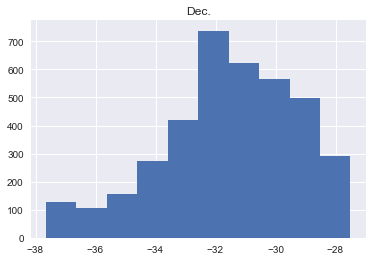In :
conv = (np.pi/180.)
r = df['V']
RA = df['R.A.']*conv
DEC   = df['Dec.']*conv
dRA = RA.max()-RA.min()
dDEC = DEC.max()-DEC.min()
color= 'darkred'
fig = plt.figure()
C = ax.scatter(RA,r,c=color,s=2,cmap='hsv',alpha=0.75)
ax.set_thetamin(RA.min()/conv)
ax.set_thetamax(RA.max()/conv)
ax.set_theta_offset(245*conv)
ax.set_rmax(25000)
ax.set_xticklabels(['','','',np.round(RA.mean()/conv),'',''])
ax.set_title('R.A.',y=1.1)
ax.text(0,3000,'Redshift [km/s]')
plt.show()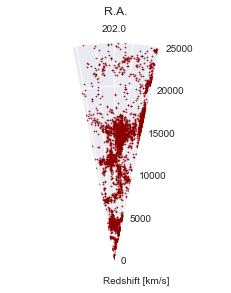In :
yRA = (RA-(RA.min()+dRA*0.5))*(np.pi/180.)
yDEC  = (DEC-(DEC.min()+dDEC*0.5))*(np.pi/180.)
rRA =r*np.sin(yRA)
rDEC=r*np.sin(yDEC)

In :
plt.scatter(rRA,r,marker='.',alpha=0.5)
plt.xlim(-100,100)
plt.ylim(0,25000)
plt.xlabel('Redshift * sin(R.A.+cst.)')
plt.ylabel('Redshift')
plt.show()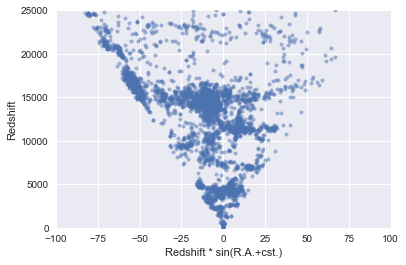In :
X = np.vstack((rRA,r))
X=X.T
Xs = StandardScaler().fit_transform(X)


#### Galaxy group clustering¶

We will explore the performance of a couple of clustering algortihms starting with the simple k-mean method. k-mean, where k is the number of clusters, can be seen as a special case of Gaussian mixture model with equal covariance per component.

k-means starts with a random choice of cluster centers. For repeatability, one should set the value of random_state.

An alternative to k-means is k-median, where the median is used instead of the mean. It has the advantage to be more robust to outliers but is is also much slower.

As criterion to find the optimal number of clusters, we will use the silhouette method, which aims to explain the consistancy within cluster data. The silhouette value will range between -1 and 1 and a high value indicates that items are well matched within clusters and weakly matched to neighboring clusters.

The best value is 1 and the worst value is -1. Values near 0 indicate overlapping clusters. Negative values generally indicate that a sample has been assigned to the wrong cluster, as a different cluster is more similar.

#### K-means¶

In :
# Python 2.7
# Scikit-learn version 0.19
# k-means algorithm
from sklearn.cluster import KMeans
#help("sklearn.cluster.KMeans")

In :
col_list = ['blue','silver','darkgoldenrod','darkgreen',
'darkmagenta','red','darkorange','gold',
'darkolivegreen','burlywood','chartreuse',
'chocolate','coral','cornflowerblue','black',
'darkkhaki','pink','moccasin','limegreen']

from matplotlib import cm
# Use all colors that matplotlib provides by default.
color = cycle(colors.cnames.keys())
color_iter = cycle(col_list)

def plot_clustering(title):
plt.subplots(figsize=(8, 5))
plt.subplot(111)
plt.scatter(rRA,r,alpha=0.5,marker=".")
plt.title(title)
for i in range(n_clusters_):
color = cm.spectral(float(i) / n_clusters)
plt.scatter(rRA[labels == i],r[labels == i],
alpha=0.5,marker=".",color=color)
plt.xlabel('Redshift * sin(R.A.+cst.)')
plt.ylabel('Redshift (km/s)')

In :
from sklearn.metrics import silhouette_score, silhouette_samples
from sklearn.cluster import KMeans

all_scores = []
clusters = np.arange(5,12,1)
for n_clusters in clusters:
kmodel = KMeans(n_clusters=n_clusters,random_state=1)
kmodel.fit(Xs)
labels = kmodel.labels_
centroids = kmodel.cluster_centers_
score = silhouette_score(Xs, labels, metric='sqeuclidean')
all_scores.append(score)
print "Number of clusters:",n_clusters,"Silhouette score: %0.3f" % score
# Set the size of the plot
plt.figure(figsize=(10,4))
plt.subplot(1, 2, 1)
plt.plot(clusters,all_scores)
plt.grid(True)
plt.ylabel("Silhouette Score")
plt.xlabel("k")
plt.title("Silhouette for K-means")

ibest = np.argmax(all_scores)
print "Choosing n_clusters=", clusters[ibest]
# Initialize the clusterer with n_clusters value and a random generator
model = KMeans(n_clusters=clusters[ibest], init='k-means++',
n_init=10, random_state=1)
model.fit_predict(Xs)
cluster_labels = np.unique(model.labels_)
n_clusters = cluster_labels.shape

# Compute the silhouette scores for each sample
silhouette_vals = silhouette_samples(X, model.labels_)

plt.subplot(1, 2, 2)

y_lower, y_upper = 0,0
yticks = []
for i, c  in enumerate(cluster_labels):
c_silhouette_vals = silhouette_vals[model.labels_ ==c]
c_silhouette_vals.sort()
y_upper += len(c_silhouette_vals)
color = cm.spectral(float(i) / n_clusters)
plt.barh(range(y_lower, y_upper), c_silhouette_vals,
facecolor=color,
edgecolor=color, alpha=0.7)
yticks.append((y_lower + y_upper) / 2)
y_lower += len(c_silhouette_vals)
silhouette_avg = np.mean(silhouette_vals)

plt.yticks(yticks, cluster_labels+1)
# The vertical line for average silhouette score of all the values
plt.axvline(x=silhouette_avg, color="red", linestyle="--")
plt.ylabel('Cluster')
plt.xlabel('Silhouette coefficient')
plt.title("Silhouette for K-means")
plt.show()

# we choose the number of clusters to be 8
n_clusters_ = clusters[ibest]
k_means = KMeans(n_clusters=n_clusters_,random_state=1)
k_means.fit(Xs)
labels = k_means.labels_
print("Silhouette Coefficient: %0.3f"
% silhouette_score(Xs, labels, metric='sqeuclidean'))
plot_clustering('k-means')

Number of clusters: 5 Silhouette score: 0.669
Number of clusters: 6 Silhouette score: 0.668
Number of clusters: 7 Silhouette score: 0.678
Number of clusters: 8 Silhouette score: 0.714
Number of clusters: 9 Silhouette score: 0.695
Number of clusters: 10 Silhouette score: 0.662
Number of clusters: 11 Silhouette score: 0.657
Choosing n_clusters= 8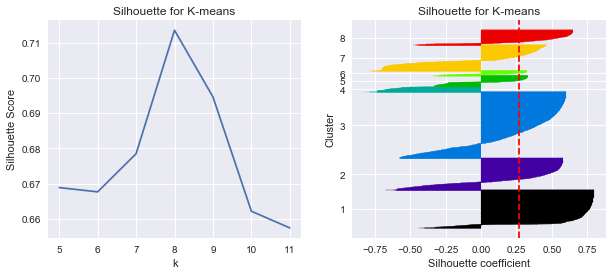Silhouette Coefficient: 0.714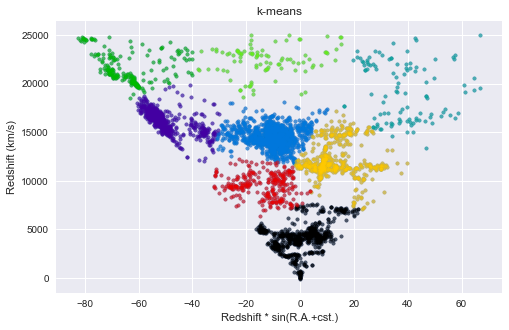#### k-Medians¶

A simple alternative to k-Means is to use the median instead of the mean values.

In :
# require sklearn-extensions
# (sudo) pip install sklearn-extensions
from sklearn_extensions.fuzzy_kmeans import KMedians

n_clusters_ = clusters[ibest]
k_median = KMedians(k=n_clusters_,random_state=1)
k_median.fit(Xs)
labels = k_median.labels_
print("Silhouette Coefficient: %0.3f"
% silhouette_score(Xs, labels, metric='sqeuclidean'))
plot_clustering('k-median')

Silhouette Coefficient: 0.479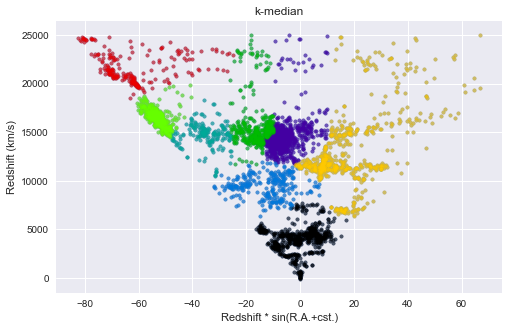#### Expectation-Maximixation (EM) clustering using Gaussian Mixture Models (GMM)¶

K-means use a simple definition for the cluster centers. K-means performs well for clusters in the form of blobs. Using GMM means that we have that the data points are gaussian distrubuted. In 2D clusters can then take ellipses as shape. In high-dimension the shapes are still ellipsoids. In this method each gaussian is assigned a cluster. The parameters of the Gaussians are found by the expectation-maximization optimization method. In contrast to other clustering methods, a data point can belong to more than one cluster, this is called a mixed membership.

In :
from sklearn.mixture import GaussianMixture
#help("sklearn.mixture.GaussianMixture")

In :
lowest_bic = np.infty
bic = []
n_components_range = range(1, 15)
cv_types = ['spherical', 'tied', 'diag', 'full']
for cv_type in cv_types:
for n_components in n_components_range:
#Fit a Gaussian mixture with EM
gmm = GaussianMixture(n_components=n_components,
covariance_type=cv_type,tol=1e-3,max_iter=150,
n_init=1,random_state=2018)
gmm.fit(Xs)
bic.append(gmm.bic(Xs))
if bic[-1] < lowest_bic:
lowest_bic = bic[-1]
best_gmm = gmm # save the model with the lowest BIC
nbest = n_components

bic = np.array(bic)
bars = []
print "Best model number of components:",nbest

Best model number of components: 13

In :
# Plot the BIC scores
plt.subplots(figsize=(12, 5))
for i, (cv_type, color) in enumerate(zip(cv_types, color_iter)):
xpos = np.array(n_components_range) + .2 * (i - 2)
bars.append(plt.bar(xpos, bic[i * len(n_components_range):
(i + 1) * len(n_components_range)],
width=.2, color=color))

plt.xticks(n_components_range)
plt.ylim([bic.min() * 1.01 - .01 * bic.max(), bic.max()])
plt.title('BIC score per model')
xpos = np.mod(bic.argmin(), len(n_components_range)) + .65 +\
.2 * np.floor(bic.argmin() / len(n_components_range))
plt.text(xpos, bic.min() * 0.97 + .03 * bic.max(), '*', fontsize=14)
plt.xlabel('Number of components')
plt.legend([b for b in bars], cv_types)
plt.show()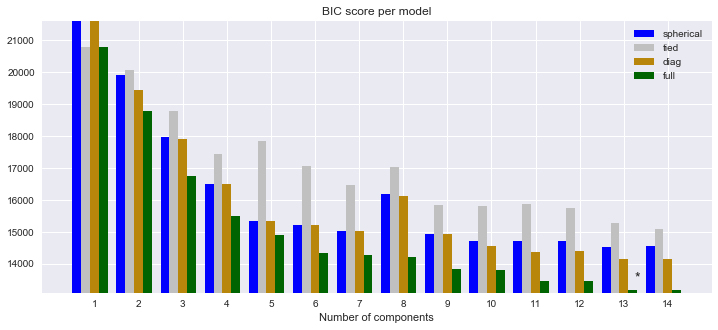In :
# Plot the best solution
splot= plt.subplots(figsize=(8, 5))
splot = plt.subplot(111)
Y_ = best_gmm.predict(Xs)

def plot_gmm():
for i, (mean, cov, color) in enumerate(zip(best_gmm.means_,
best_gmm.covariances_,
color_iter)):
v, w = np.linalg.eigh(cov)
if not np.any(Y_ == i):
continue
plt.scatter(Xs[Y_ == i, 0], Xs[Y_ == i, 1],
alpha=0.5, color=color,marker=".")
# Plot an ellipse to show the Gaussian component
angle = np.arctan2(w, w)
angle = 180. * angle / np.pi # convert to degrees
v = 2. * np.sqrt(2.) * np.sqrt(v)
ell = mpl.patches.Ellipse(mean,
v, v, 180. + angle, color=color)
ell.set_clip_box(splot.bbox)
ell.set_alpha(.6)

plt.xlabel('Normlaized Redshift x sin(R.A.+cst.)')
plt.ylabel('Normalized Redshift')
plt.title('Selected Gaussian Mixture: full model')
plt.show()
plot_gmm()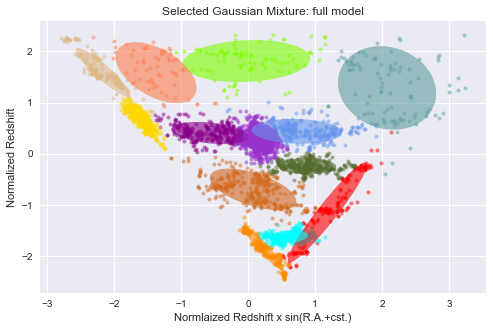In :
# DBSCAN algorithm
from sklearn.cluster import DBSCAN
#help("sklearn.cluster.DBSCAN")
# DBSCAN - Density-Based Spatial Clustering of Applications with Noise.
#  Finds core samples of high density and expands clusters from them.
#  Good for data which contains clusters of similar density.

In :
db = DBSCAN(eps=0.1,min_samples=10).fit(Xs)
labels = db.labels_
# Number of clusters in labels, ignoring noise if present.
n_clusters_ = len(set(labels)) - (1 if -1 in labels else 0)
print('Estimated number of clusters: %d' % n_clusters_)
print("Silhouette Coefficient: %0.3f"
% silhouette_score(Xs, labels, metric='sqeuclidean'))

Estimated number of clusters: 11
Silhouette Coefficient: 0.190

In :
plot_clustering("DBSCAN")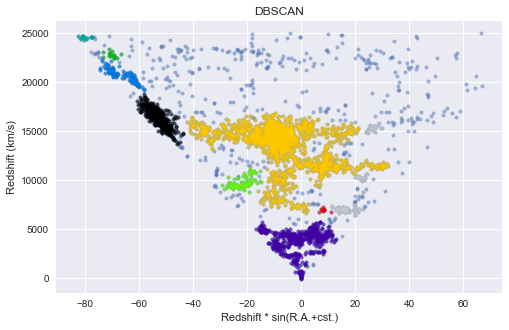DBSCAN algorithm is based on density. Therefore it tends to find clusters where there is gathering of galaxies. Galaxies in low density regions belong to any cluster. The algorithm can identify outliers unlike the mean-shift method and esclude them from the clusters. As such DBSCAN can be used to find outliers. It can find arbitrary sized and shaped clusters.

The main limitation is that DBSCAN is not performing well when clusters of different sizes are present because of the usage of a threshold distance and minmum points for identifying neighbors will then vary for cluster to cluster.

In our example, DBSCAN has excluded high redshift galaxies as cluster members because of the distances that separate each of them from the others.

#### Agglomerative hierarchical clustering¶

Agglomerative hierarchical clustering is based on a Tree algorithm. This algorithm is called in astronomy the friends-of-friends algorihtm.

In :
from sklearn.cluster import AgglomerativeClustering
#help("sklearn.cluster.AgglomerativeClustering")

In :
# Recursively merges the pair of clusters that minimally increases
# linkage : {"ward", "complete", "average"}, optional, default: "ward"
# affinity : string or callable, default: "euclidean"
#            Metric used to compute the linkage.
#            Can be "euclidean", "l1", "l2","manhattan", "cosine", or
#            'precomputed'.
#            If linkage is "ward", only "euclidean" is accepted.
n_clusters_ = 10
ACmodel = AgglomerativeClustering(n_clusters=n_clusters_,
ACmodel.fit(Xs)
labels = ACmodel.labels_
print("Silhouette Coefficient: %0.3f"
% silhouette_score(Xs, labels, metric='sqeuclidean'))
plot_clustering("Agglomerative Clustering (euclidean, average)")

Silhouette Coefficient: 0.613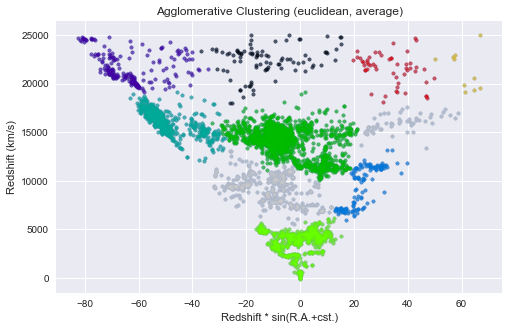In :
n_clusters_ = 10
ACmodel = AgglomerativeClustering(n_clusters=n_clusters_,
ACmodel.fit(Xs)
labels = ACmodel.labels_
print("Silhouette Coefficient: %0.3f"
% silhouette_score(Xs, labels, metric='sqeuclidean'))
plot_clustering("Agglomerative Clustering (euclidean, ward)")

Silhouette Coefficient: 0.610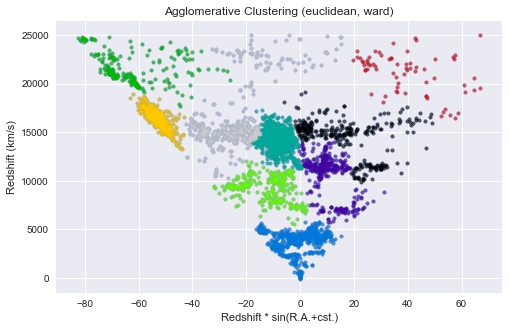In :
n_clusters_ = 10
ACmodel = AgglomerativeClustering(n_clusters=n_clusters_,
ACmodel.fit(Xs)
labels = ACmodel.labels_
print("Silhouette Coefficient: %0.3f"
% silhouette_score(Xs, labels, metric='sqeuclidean'))
plot_clustering("Agglomerative Clustering (Manhattan, average)")

Silhouette Coefficient: 0.601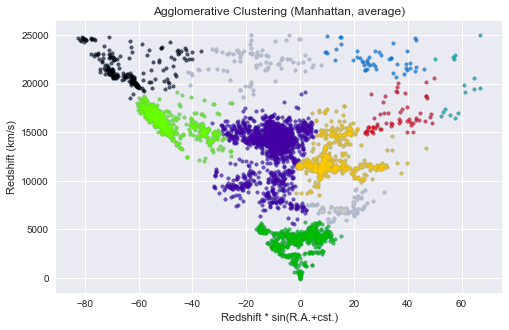In :
# mean-shift clustering algorithm
from sklearn.cluster import MeanShift, estimate_bandwidth
#help("sklearn.cluster.MeanShift")
#help("sklearn.cluster.estimate_bandwidth")

In :
# Compute clustering with MeanShift
# The following bandwidth can be automatically detected using
bandwidth = estimate_bandwidth(Xs, quantile=0.1, n_samples=200)
ms = MeanShift(bandwidth=bandwidth, bin_seeding=True,
cluster_all=False)
ms.fit(Xs)
labels = ms.labels_
cluster_centers = ms.cluster_centers_
labels_unique = np.unique(labels)
n_clusters_ = len(labels_unique)
print("number of estimated clusters : %d" % n_clusters_)
print("Silhouette Coefficient: %0.3f"
% silhouette_score(Xs, labels, metric='sqeuclidean'))
plot_clustering("Mean Shift")

number of estimated clusters : 9
Silhouette Coefficient: 0.399